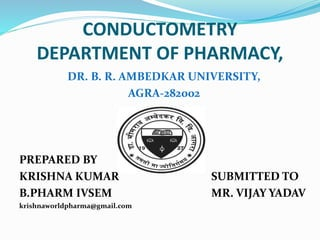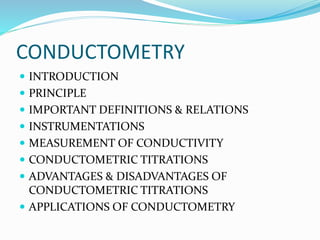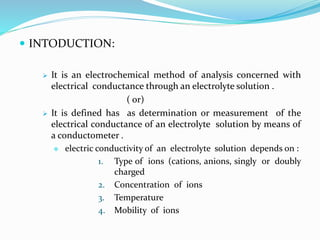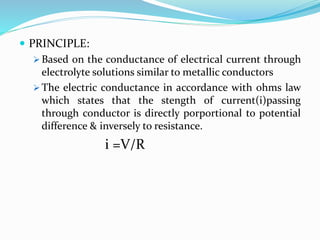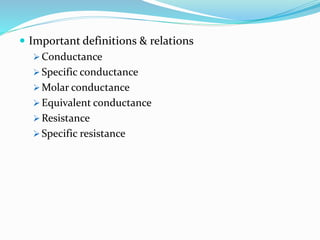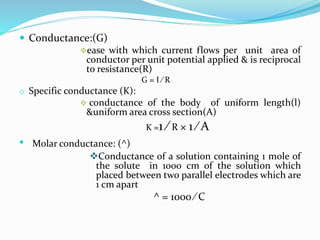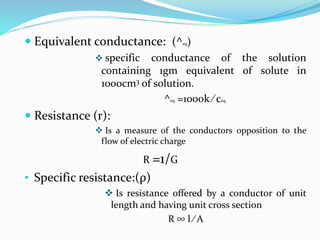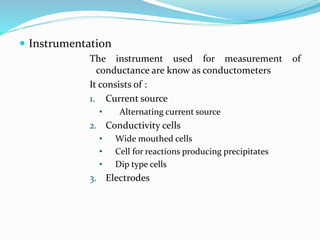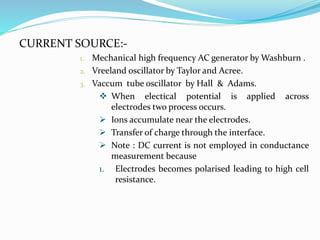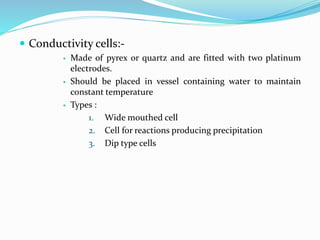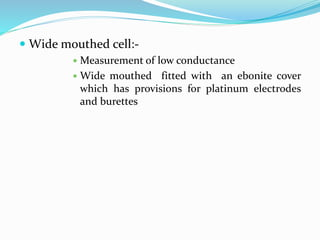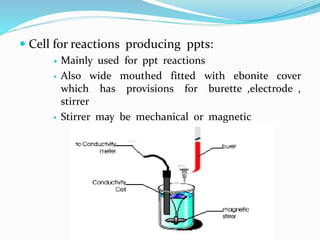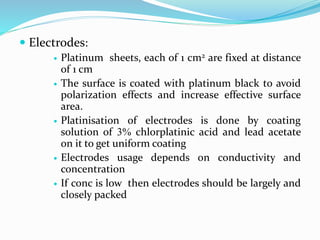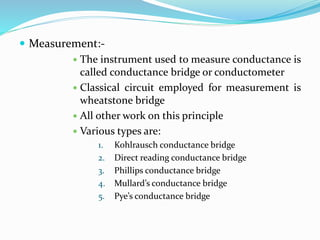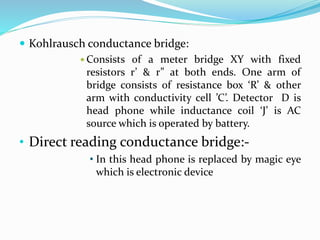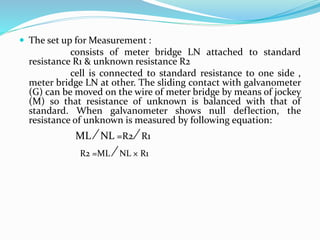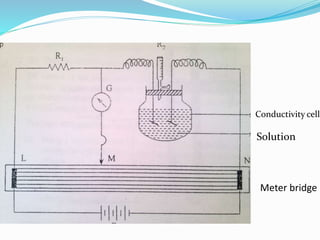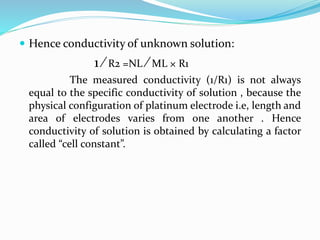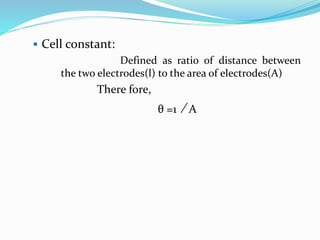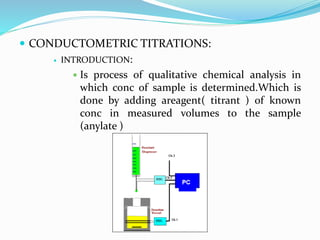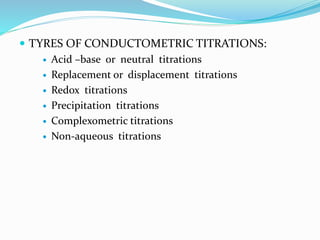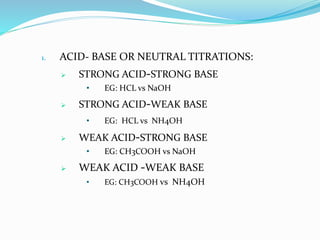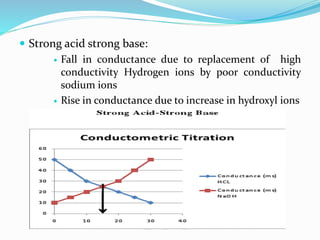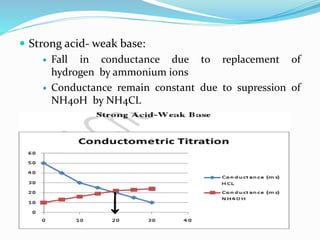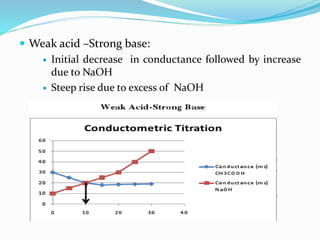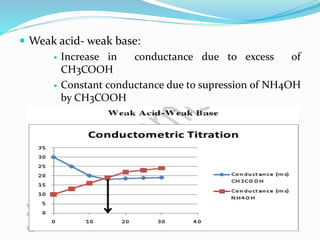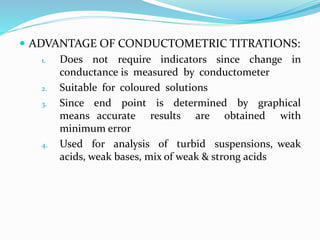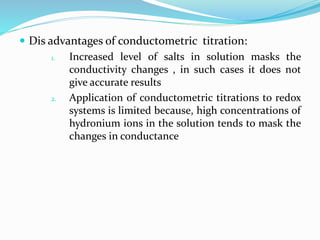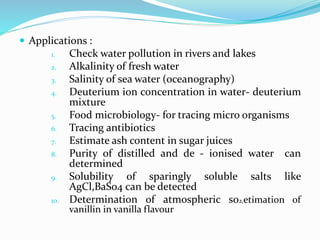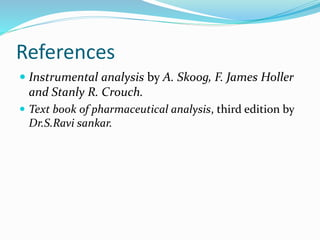1 of 32

### conductometry-170513191040.pdf

1. CONDUCTOMETRY DEPARTMENT OF PHARMACY, DR. B. R. AMBEDKAR UNIVERSITY, AGRA-282002 PREPARED BY KRISHNA KUMAR SUBMITTED TO B.PHARM IVSEM MR. VIJAY YADAV krishnaworldpharma@gmail.com
2. CONDUCTOMETRY  INTRODUCTION  PRINCIPLE  IMPORTANT DEFINITIONS & RELATIONS  INSTRUMENTATIONS  MEASUREMENT OF CONDUCTIVITY  CONDUCTOMETRIC TITRATIONS  ADVANTAGES & DISADVANTAGES OF CONDUCTOMETRIC TITRATIONS  APPLICATIONS OF CONDUCTOMETRY
3.  INTODUCTION:  It is an electrochemical method of analysis concerned with electrical conductance through an electrolyte solution . ( or)  It is defined has as determination or measurement of the electrical conductance of an electrolyte solution by means of a conductometer .  electric conductivity of an electrolyte solution depends on : 1. Type of ions (cations, anions, singly or doubly charged 2. Concentration of ions 3. Temperature 4. Mobility of ions
4.  PRINCIPLE:  Based on the conductance of electrical current through electrolyte solutions similar to metallic conductors  The electric conductance in accordance with ohms law which states that the stength of current(i)passing through conductor is directly porportional to potential difference & inversely to resistance. i =V/R
5.  Important definitions & relations  Conductance  Specific conductance  Molar conductance  Equivalent conductance  Resistance  Specific resistance
6.  Conductance:(G) ease with which current flows per unit area of conductor per unit potential applied & is reciprocal to resistance(R) G = I ⁄ R o Specific conductance (K):  conductance of the body of uniform length(l) &uniform area cross section(A) K =1 ⁄ R × 1 ⁄ A • Molar conductance: (^) Conductance of a solution containing 1 mole of the solute in 1000 cm of the solution which placed between two parallel electrodes which are 1 cm apart ^ = 1000 ⁄ C
7.  Equivalent conductance: (^eq)  specific conductance of the solution containing 1gm equivalent of solute in 1000cm3 of solution. ^eq =1000k ⁄ ceq  Resistance (r):  Is a measure of the conductors opposition to the flow of electric charge R =1/G • Specific resistance:(ρ)  Is resistance offered by a conductor of unit length and having unit cross section R ∞ l ⁄ A
8.  Instrumentation The instrument used for measurement of conductance are know as conductometers It consists of : 1. Current source • Alternating current source 2. Conductivity cells • Wide mouthed cells • Cell for reactions producing precipitates • Dip type cells 3. Electrodes
9. CURRENT SOURCE:- 1. Mechanical high frequency AC generator by Washburn . 2. Vreeland oscillator by Taylor and Acree. 3. Vaccum tube oscillator by Hall & Adams.  When electical potential is applied across electrodes two process occurs.  Ions accumulate near the electrodes.  Transfer of charge through the interface.  Note : DC current is not employed in conductance measurement because 1. Electrodes becomes polarised leading to high cell resistance.
10.  Conductivity cells:-  Made of pyrex or quartz and are fitted with two platinum electrodes.  Should be placed in vessel containing water to maintain constant temperature  Types : 1. Wide mouthed cell 2. Cell for reactions producing precipitation 3. Dip type cells
11.  Wide mouthed cell:-  Measurement of low conductance  Wide mouthed fitted with an ebonite cover which has provisions for platinum electrodes and burettes
12.  Cell for reactions producing ppts:  Mainly used for ppt reactions  Also wide mouthed fitted with ebonite cover which has provisions for burette ,electrode , stirrer  Stirrer may be mechanical or magnetic
13.  Electrodes:  Platinum sheets, each of 1 cm2 are fixed at distance of 1 cm  The surface is coated with platinum black to avoid polarization effects and increase effective surface area.  Platinisation of electrodes is done by coating solution of 3% chlorplatinic acid and lead acetate on it to get uniform coating  Electrodes usage depends on conductivity and concentration  If conc is low then electrodes should be largely and closely packed
14.  Measurement:-  The instrument used to measure conductance is called conductance bridge or conductometer  Classical circuit employed for measurement is wheatstone bridge  All other work on this principle  Various types are: 1. Kohlrausch conductance bridge 2. Direct reading conductance bridge 3. Phillips conductance bridge 4. Mullard’s conductance bridge 5. Pye’s conductance bridge
15.  Kohlrausch conductance bridge:  Consists of a meter bridge XY with fixed resistors r’ & r” at both ends. One arm of bridge consists of resistance box ‘R’ & other arm with conductivity cell ’C’. Detector D is head phone while inductance coil ‘J’ is AC source which is operated by battery. • Direct reading conductance bridge:- • In this head phone is replaced by magic eye which is electronic device
16.  The set up for Measurement : consists of meter bridge LN attached to standard resistance R1 & unknown resistance R2 cell is connected to standard resistance to one side , meter bridge LN at other. The sliding contact with galvanometer (G) can be moved on the wire of meter bridge by means of jockey (M) so that resistance of unknown is balanced with that of standard. When galvanometer shows null deflection, the resistance of unknown is measured by following equation: ML ⁄ NL =R2 ⁄ R1 R2 =ML ⁄ NL × R1
17. Conductivity cell Solution Meter bridge
18.  Hence conductivity of unknown solution: 1 ⁄ R2 =NL ⁄ ML × R1 The measured conductivity (1/R1) is not always equal to the specific conductivity of solution , because the physical configuration of platinum electrode i.e, length and area of electrodes varies from one another . Hence conductivity of solution is obtained by calculating a factor called “cell constant”.
19.  Cell constant: Defined as ratio of distance between the two electrodes(l) to the area of electrodes(A) There fore, θ =1 ⁄ A
20.  CONDUCTOMETRIC TITRATIONS:  INTRODUCTION:  Is process of qualitative chemical analysis in which conc of sample is determined.Which is done by adding areagent( titrant ) of known conc in measured volumes to the sample (anylate )
21.  TYRES OF CONDUCTOMETRIC TITRATIONS:  Acid –base or neutral titrations  Replacement or displacement titrations  Redox titrations  Precipitation titrations  Complexometric titrations  Non-aqueous titrations
22. 1. ACID- BASE OR NEUTRAL TITRATIONS:  STRONG ACID-STRONG BASE • EG: HCL vs NaOH  STRONG ACID-WEAK BASE • EG: HCL vs NH4OH  WEAK ACID-STRONG BASE • EG: CH3COOH vs NaOH  WEAK ACID -WEAK BASE • EG: CH3COOH vs NH4OH
23.  Strong acid strong base:  Fall in conductance due to replacement of high conductivity Hydrogen ions by poor conductivity sodium ions  Rise in conductance due to increase in hydroxyl ions
24.  Strong acid- weak base:  Fall in conductance due to replacement of hydrogen by ammonium ions  Conductance remain constant due to supression of NH40H by NH4CL
25.  Weak acid –Strong base:  Initial decrease in conductance followed by increase due to NaOH  Steep rise due to excess of NaOH
26.  Weak acid- weak base:  Increase in conductance due to excess of CH3COOH  Constant conductance due to supression of NH4OH by CH3COOH
27.  ADVANTAGE OF CONDUCTOMETRIC TITRATIONS: 1. Does not require indicators since change in conductance is measured by conductometer 2. Suitable for coloured solutions 3. Since end point is determined by graphical means accurate results are obtained with minimum error 4. Used for analysis of turbid suspensions, weak acids, weak bases, mix of weak & strong acids
28.  Dis advantages of conductometric titration: 1. Increased level of salts in solution masks the conductivity changes , in such cases it does not give accurate results 2. Application of conductometric titrations to redox systems is limited because, high concentrations of hydronium ions in the solution tends to mask the changes in conductance
29.  Applications : 1. Check water pollution in rivers and lakes 2. Alkalinity of fresh water 3. Salinity of sea water (oceanography) 4. Deuterium ion concentration in water- deuterium mixture 5. Food microbiology- for tracing micro organisms 6. Tracing antibiotics 7. Estimate ash content in sugar juices 8. Purity of distilled and de - ionised water can determined 9. Solubility of sparingly soluble salts like AgCl,BaSo4 can be detected 10. Determination of atmospheric so2,etimation of vanillin in vanilla flavour
30. References  Instrumental analysis by A. Skoog, F. James Holler and Stanly R. Crouch.  Text book of pharmaceutical analysis, third edition by Dr.S.Ravi sankar.
31. Presented by KRISHNA KUMAR THE END